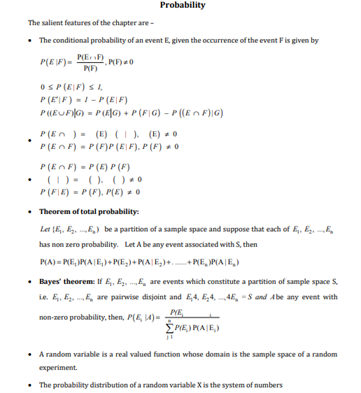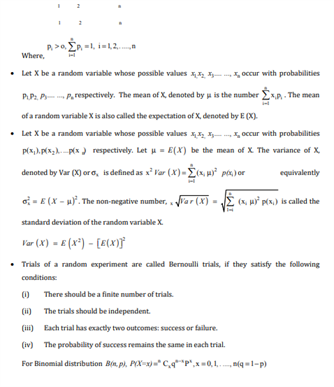# Maths Revision Notes for Class 12 Chapter 13 Probability.

The 13th chapter in the CBSE class 12 Maths revision notes is titled as Probability. Students can refer to these revision notes for the chapter of Probability while revising for their main exams. Simply put, Probability can be defined as the likeliness or extent to which a particular event might take place. The extent of probability is usually grouped between 0 and 1, where 0 indicates the impossibility of an event, while 1 expresses the certainty of an event. Some of the other concepts that students will learn in this chapter are:

• Basic definitions of a random experiment, sample-space, experiment or trial and event.
• Besides these definitions, the classification of events is also described along with the difference between a simple event and a compound event.
• There are other cases of events which might also occur such as a mutually exclusive event, independent events and exhaustive events.

Students can refer to the revision notes of chapter 13 Probability given below:You may also want to read:

 NCERT Related Articles NCERT Solutions for Class 12 NCERT Solutions for Class 11 NCERT Solutions for Class 10 NCERT Solutions for Class 12 Maths NCERT Solutions for Class 11 Maths NCERT Solutions for Class 10 Maths NCERT Solutions for Class 12 Physics NCERT Solutions for Class 11 Physics NCERT Solutions for Class 10 Science NCERT Solutions for Class 12 Chemistry NCERT Solutions for Class 11 Chemistry NCERT Solutions for Class 6 NCERT Solutions for Class 12 Biology NCERT Solutions for Class 11 Biology NCERT Solutions for Class 6 Maths NCERT Solutions for Class 9 NCERT Solutions for Class 8 NCERT Solutions for Class 6 Science NCERT Solutions for Class 9 Maths NCERT Solutions for Class 8 Maths NCERT Solutions for Class 4 NCERT Solutions for Class 9 Science NCERT Solutions for Class 8 Science NCERT Solutions for Class 4 Maths NCERT Solutions for Class 5 NCERT Solutions for Class 7 NCERT Solutions for Class 7 Maths NCERT Solutions for Class 4 Science NCERT Solutions for Class 5 Maths NCERT Solutions for Class 7 Science NCERT Solutions NCERT Books NCERT Solutions for Class 5 Science